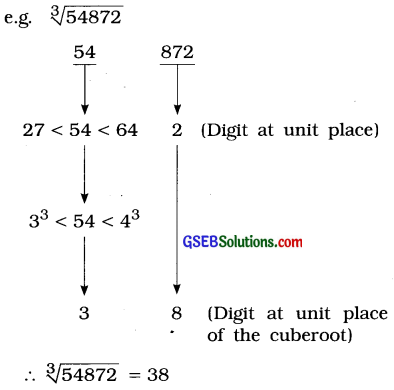# GSEB Class 8 Maths Notes Chapter 7 Cubes and Cube Roots

This GSEB Class 8 Maths Notes Chapter 7 Cubes and Cube Roots covers all the important topics and concepts as mentioned in the chapter.

## Cubes and Cube Roots Class 8 GSEB Notes

→ Taking one and the same number three times and multiplying, we get the cube of that number. In other words, repeated multiplication of a number three times gives the cube of that number,
e.g. 1 × 1 × 1 = 13 = 1
2 × 2 × 2 = 23 = 8
3 × 3 × 3 = 33 = 27

→ Remember that the cube of 1 is 1, the cube of 2 is 8 and the cube of 3 is 27. Moreover, the cube root of 1 is 1, the cube root of 8 is 2 and the cube root of 27 is 3.

→ Symbolically:
$$\sqrt{1}$$ = 1. Read as cube root of 1 is equal to 1
$$\sqrt{8}$$ = 2. Read as cube root of 8 is equal to 2
$$\sqrt{27}$$ = 3. Read as cube root of 27 is equal to 3
Thus, 1, 8, 27, 64, 125,… are positive perfect cubes as they are the cubes of natural numbers.

→ Among the numbers from 1 to 100, there are four perfect cubes 1, 8, 27 and 64.

→ Every natural number has a cube, but every natural number does not have a cube root.

→ Definition: The cube of any integer is called a perfect cube.

→ Multiplying the square of a number by the number, we get the cube of that number.
e.g. The square of 2 is 4. Multiplying 4 by 2,
i. e., 4 × 2 = 8 which is the cube of 2, and so on.
(Note: Cubes of odd numbers are always odd while cubes of even numbers are always even. ]

→ If the digit at units place of a number is 1, 4, 5, 6, 9 or 0, the digit at units place of the cube of that number is also the same digit, i.e., 1, 4, 5, 6, 9 or 0 respectively.

→ If the digit at units place of a number is 2, the digit at units place of the cube of that number is 8.

→ If the digit at units place of a number is 8, the digit at units place of the cube of that number is 2.

→ If the digit at units place of a number is 3, the digit at units place of the cube of that number is 7.

→ If the digit at units place of a number is 7, the digit at units place of the cube of that number is 3.

→ If the digit at units place of a number is 0, last three digits of the cube of that number are zeros.
e.g. 103 = 10 × 10 × 10 = 1000
203 = 20 × 20 × 20 = 8000
303 = 30 × 30 × 30 = 27,000
403 = 40 × 40 × 40 = 64,000→ Hardy – Ramanujan Numbers :
Numbers which can be expressed as a sum of two cubes in two different ways are called Hardy – Ramanujan Numbers,
e.g. 1729 = 1728 + 1 = 123 + 13, and
1729 = 1000 + 729 = 103 + 93

→ 1729 is called a ‘dull number’.

→ There are an infinitely many such numbers. Few are 4104 (2, 16; 9, 15), 13832 (18, 20; 2, 24) and so on.

→ The cube root of any perfect cube made up of at the most six digits can easily be found by logical inference (estimation).Explanation:

• Split the given number into two parts :
1. The number formed by the digits at the hundreds, tens and units place of the given number. Here, it is 872.
2. The number formed by the remaining digits in the same order. Here, it is 54.
• In the first part 872, the digit at units place is 2, which gives 8 at the units place of the cube root.
• Find two consecutive perfect cubes between which the number in the second part lies. Here, the number in the second part, i.e., 54 lies between 27 and 64. Thus, 33 < 54 < 43. Now, 54 is greater than the cube of 3. Hence, the digit at tens place of the cube root of the given number is 3.
∴ $$\sqrt{54872}$$ = 38

→ Similarly, $$\sqrt{148877}$$ = 53 as 53 < 148 < 63 and 7 at units place of the perfect cube gives 3 at the units place of the cube root.